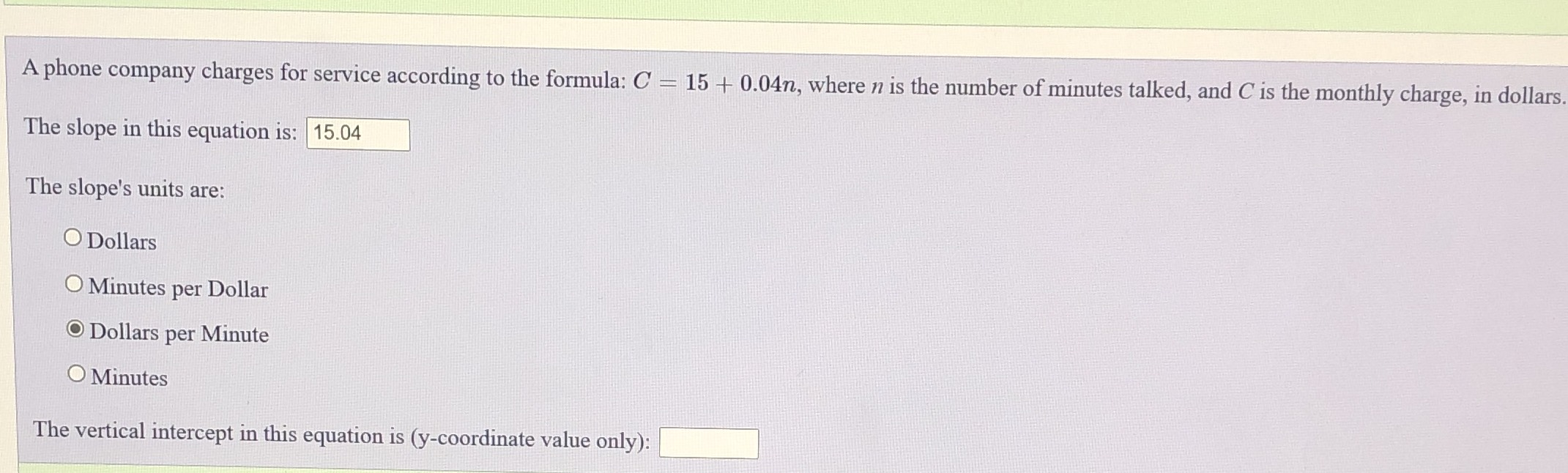### ¿Todavía tienes preguntas de matemáticas?

Pregunte a nuestros tutores expertos
Algebra
PreguntaA phone company charges for service according to the formula: $$C = 15 + 0.04 n$$ , where $$n$$ is the number of minutes talked, and $$C$$ is the monthly charge, in dollars. The slope in this equation is: $$\square$$

The slope's units are:

Dollars

Minutes per Dollar

Dollars per Minute

Minutes

The vertical intercept in this equation is $$( y$$ -coordinate value only): $$\square$$.